Stat 330 Assignment 10 Partial Solutions

1. Chapter 12 Q2: When the accelerated strength is x the regression model says that 28 day strength is normally distributed with SD 350 and mean 1800+1.3x.

1. For x=2000 the mean becomes 4400. The probability that 28 day strength is more than 5000 is the probability that a standard normal variate is more than (5000-4400)/350=1.71. This probability is 0.0436.
2. For x=2500 the mean is 5050 and the probability is the area to the right of -50/350 which is 0.556.
3. Let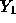be the observation at x=2500 and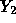the observation x=2000. Then the mean of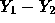is 1.3(500)=650 while the SD of the difference is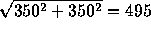. The probability we want is that a standard normal is more than (1000-650)/495=0.707.This is roughly 0.24.
4. Now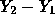is normal with mean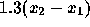and SD 495. We want the probability that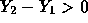or the probability to the right of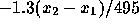to be 0.95. This means that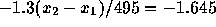or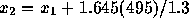. The answer is then 626.

2. Q16: I used the following SAS code and ran the code twice with and without the last point.
```options pagesize =60 linesize=80;
data q16;
infile 'q16.dat';
input x y;
proc reg;
model y=x;
plot residual.*predicted.;
plot y*x;
run;```
The output for the full data set is
```Dependent Variable: Y
Analysis of Variance
Sum of         Mean
Source          DF      Squares       Square      F Value       Prob>F
Model            1 538208.57051 538208.57051      385.024       0.0001
Error            4   5591.42949   1397.85737
C Total          5 543800.00000

Root MSE      37.38793     R-square       0.9897
Dep Mean     560.00000     Adj R-sq       0.9871
C.V.           6.67642

Parameter Estimates

Parameter      Standard    T for H0:
Variable  DF      Estimate         Error   Parameter=0    Prob > |T|

INTERCEP   1    137.875631   26.37756553         5.227        0.0064
X          1      9.311567    0.47454663        19.622        0.0001

----+-----+-----+-----+-----+-----+-----+-----+-----+-----+-----+----
RESIDUAL |                                                                   |
|                                                                   |
50 +                                                                   +
|                                                                   |
|                                                                   |
|         1                                                         |
|                                                                   |
40 +                                                                   +
|                                                                   |
|                                                                   |
|                                                                   |
|                                                                   |
30 +                                                                   +
|                                                                   |
|                                                                   |
|                                                                   |
|                                                                   |
20 +                                                              1    +
|                                                                   |
|                                                                   |
|                                                                   |
R          |       1                                                           |
e       10 +                                                                   +
s          |                                                                   |
i          |                                                                   |
d          |                        1                                          |
u          |                                                                   |
a        0 +                                                                   +
l          |                                                                   |
|                                                                   |
|                                                                   |
|                                                                   |
-10 +                                                                   +
|                                                                   |
|                                                                   |
|                                                                   |
|                                                                   |
-20 +                                                                   +
|                                                                   |
|                                                                   |
|                                                                   |
|                                                                   |
-30 +                                                                   +
|                                                                   |
|                                                                   |
|                                                                   |
|                       1                                           |
-40 +                      1                                            +
|                                                                   |
|                                                                   |
----+-----+-----+-----+-----+-----+-----+-----+-----+-----+-----+----
200   300   400   500   600   700   800   900  1000  1100  1200

Predicted Value of Y          PRED

----+-----+-----+-----+-----+-----+-----+-----+-----+-----+-----+-----+----
Y |                                                                         |
|                                                                         |
|                                                                         |
|                                                                         |
|                                                                         |
1200 +                                                                1        +
|                                                                         |
|                                                                         |
|                                                                         |
1100 +                                                                         +
|                                                                         |
|                                                                         |
|                                                                         |
1000 +                                                                         +
|                                                                         |
|                                                                         |
|                                                                         |
900 +                                                                         +
|                                                                         |
|                                                                         |
|                                                                         |
800 +                                                                         +
|                                                                         |
|                                                                         |
|                                                                         |
700 +                                                                         +
|                                                                         |
|                                                                         |
|                                                                         |
600 +                                                                         +
|                                                                         |
|                        1                                                |
|                                                                         |
500 +                       1                                                 +
|                     1                                                   |
|                                                                         |
|                                                                         |
400 +                                                                         +
|                                                                         |
|        1                                                                |
|                                                                         |
300 +                                                                         +
|     1                                                                   |
|                                                                         |
|                                                                         |
200 +                                                                         +
|                                                                         |
|                                                                         |
|                                                                         |
|                                                                         |
----+-----+-----+-----+-----+-----+-----+-----+-----+-----+-----+-----+----
10    20    30    40    50    60    70    80    90    100   110   120

X```
while that for the edited data set is
```                                 Sum of         Mean
Source          DF      Squares       Square      F Value       Prob>F

Model            1  49839.81693  49839.81693       61.274       0.0043
Error            3   2440.18307    813.39436
C Total          4  52280.00000

Root MSE      28.52007     R-square       0.9533
Dep Mean     432.00000     Adj R-sq       0.9378
C.V.           6.60187

Parameter Estimates
Parameter      Standard    T for H0:
Variable  DF      Estimate         Error   Parameter=0    Prob > |T|

INTERCEP   1    190.352403   33.40167361         5.699        0.0107
X          1      7.551487    0.96470574         7.828        0.0043

-+----+----+----+----+----+----+----+----+----+----+----+----+----+--
RESIDUAL |                                                                   |
|                                                                   |
|                                                                   |
|                                                                   |
30 +                                                               1   +
|                                                                   |
|                                                                   |
|                                                                   |
|            1                                                      |
|                                                                   |
|                                                                   |
20 +                                                                   +
|                                                                   |
|                                                                   |
|                                                                   |
|                                                                   |
|                                                                   |
|                                                                   |
10 +                                                                   +
|                                                                   |
|                                                                   |
R          |                                                                   |
e          |                                                                   |
s          |                                                                   |
i          |                                                                   |
d        0 +                                                                   +
u          |                                                                   |
a          |                                                                   |
l          |                                                                   |
|                                                                   |
|                                                                   |
|                                                                   |
-10 +                                                                   +
|                                                                   |
|                                                                   |
|                                                                   |
|    1                                                      1       |
|                                                                   |
|                                                                   |
-20 +                                                                   +
|                                                                   |
|                                                     1             |
|                                                                   |
|                                                                   |
|                                                                   |
|                                                                   |
-30 +                                                                   +
|                                                                   |
|                                                                   |
|                                                                   |
-+----+----+----+----+----+----+----+----+----+----+----+----+----+--
280  300  320  340  360  380  400  420  440  460  480  500  520  540

Predicted Value of Y          PRED

-----+----+----+----+----+----+----+----+----+----+----+----+----+----+-----
600 +                                                                          +
|                                                                          |
|                                                                          |
|                                                                          |
|                                                                          |
|                                                                          |
|                                                                     1    |
550 +                                                                          +
|                                                                          |
|                                                                          |
|                                                                          |
|                                                                          |
|                                                                          |
|                                                                          |
500 +                                                                 1        +
|                                                                          |
|                                                                          |
Y |                                                                          |
|                                                           1              |
|                                                                          |
|                                                                          |
450 +                                                                          +
|                                                                          |
|                                                                          |
|                                                                          |
|                                                                          |
|                                                                          |
|                                                                          |
400 +                                                                          +
|                                                                          |
|                                                                          |
|                                                                          |
|                                                                          |
|                                                                          |
|                                                                          |
350 +               1                                                          +
|                                                                          |
|                                                                          |
|                                                                          |
|                                                                          |
|                                                                          |
|                                                                          |
300 +                                                                          +
|                                                                          |
|                                                                          |
|       1                                                                  |
|                                                                          |
|                                                                          |
|                                                                          |
250 +                                                                          +
-----+----+----+----+----+----+----+----+----+----+----+----+----+----+-----
12.5 15.0 17.5 20.0 22.5 25.0 27.5 30.0 32.5 35.0 37.5 40.0 42.5 45.0

X```

1. The scatter plot for the full data set seems reasonable.
2. The regression equation is y=9.3x+137.9.
3. This just asks for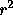but I doubt the utility of the calculation unless the x levels were set by random sampling of pairs. The value is 0.99.
4. There are many ways to compare the two lines. One is based on examining the difference in fitted slopes: 9.31 compared to 7.55 with a standard error of either about 0.47 or 1. This is not actually a significant difference assuming the model holds. Another approach is suggested in the text. The predicted value of Y when x is 112 is, for the second line 7.55(112)+190.4 or about 1036. The standard deviation of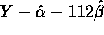is given on page 503 and you could carry out a t-test using (1200-1036)/SE and getting P-values from the t-distribution on 3 degrees of freedom.

3. Q18: You are asked to verify that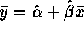. The right hand side is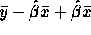which is evidently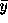.
4. Q28: The edited sas output is
```                                 Sum of         Mean
Source          DF      Squares       Square      F Value       Prob>F

Model            1      8.17906      8.17906      674.982       0.0001
Error            8      0.09694      0.01212
C Total          9      8.27600

Root MSE       0.11008     R-square       0.9883
Dep Mean       3.92000     Adj R-sq       0.9868
C.V.           2.80814

Parameter Estimates

Parameter      Standard    T for H0:
Variable  DF      Estimate         Error   Parameter=0    Prob > |T|

INTERCEP   1      2.141648    0.07679262        27.889        0.0001
X          1      0.006801    0.00026176        25.980        0.0001```

1. The output gives the estimated standard error of the slope as 0.077 and the square root of the mean squared error as 0.11.
2. The test is based on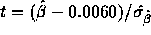which is 3.06. You get a P-value from t tables on 8 degrees of freedom and conclude that the slope is not 0.0060 (P < 0.02 two tailed).

5. Q32: We have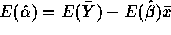(in my notation). You are allowed to assume that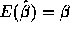. Now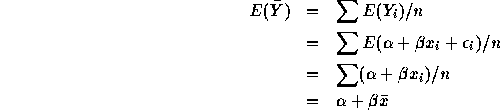Putting the two pieces we get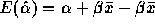which is just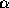.

6. Q34: Let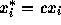and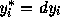. The fitted slope based on the starred data is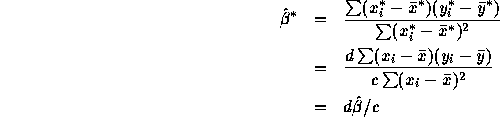The estimated standard error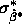of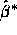is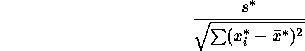To evaluate this note first that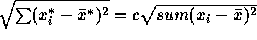. Next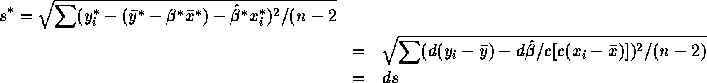Assembling the pieces shows that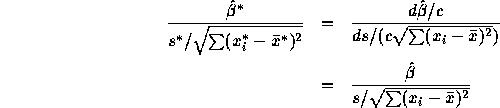7. Q52:

1. The test statistic is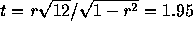on 12 degrees of freedom. For a two sided test I get P a bit over 0.10 and conclude that there is only quite weak evidence of a correlation between content and gas porosity.
2. The percent variance explained is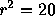%.

8. Q56: Since P is less than 0.001 we would reject the null at the level 0.001 and conclude that the correlation is not 0. However, with such a large n it takes only a quite small correlation to produce a P value of 0.00032. (To be precise: the estimated value of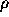must be either 0.16 or -0.16 which is a pretty weak correlation.)
9. Q58: The edited SAS output is
```                                 Sum of         Mean
Source          DF      Squares       Square      F Value       Prob>F

Model            1     25.62223     25.62223       17.604       0.0057
Error            6      8.73277      1.45546
C Total          7     34.35500

Root MSE       1.20643     R-square       0.7458
Dep Mean      77.72500     Adj R-sq       0.7034
C.V.           1.55217

Parameter Estimates

Parameter      Standard    T for H0:
Variable  DF      Estimate         Error   Parameter=0    Prob > |T|

INTERCEP   1     81.173057    0.92589886        87.669        0.0001
X          1     -0.133258    0.03176040        -4.196        0.0057```

1. The fitted line is y = -0.133x + 81.17.
2. This question asks you to test the hypothesis that the true slope is 0. The P value is 0.0057 from the output so that the hypothesis of no relation between x and Y is rejected.
3. The standard error of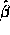is inversely proportional to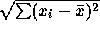. Putting 4 data points at 0 and 4 at 50 makes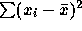equal to 5000 while the value for the data set is only about 1450. Thus the new design estimatesmore precisely. Using only 3 points at 0 and at 50 gives a sum of 3750 which is still much more precise than the design used.
4. You are supposed to plug 25 into the regression equation and then use the formulas on page 503 to attach a standard error to this number. You get 77.85 with an estimated standard error of 0.428. This does not really answer the question about whether or not efficiency has been precisely estimated but that seems to be something of a judgement.

10. Q62: I used SAS to get
```                        General Linear Models Procedure
R-Square             C.V.        Root MSE               Y Mean
0.070002         26.22198       198.15080            755.66667

T for H0:    Pr > |T|   Std Error of
Parameter                  Estimate    Parameter=0                Estimate

INTERCEPT               684.4057037           5.78     0.0007    118.3236387
X                        14.8804795           0.73     0.4915     20.5000646```
The test statistic is 0.73 with 7 degrees of freedom. Since the test is one sided we get a P value of 0.4915/2 which is certainly not significant. Thus it seems quite possible that there is no (linear) relation between eye weight and thickness.
11. Q67:

1. Since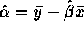it clearly suffices to check that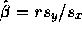. But this last value is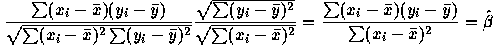as required.

2. You need to compute r and that's the answer except that if r is negative then for each SD below average on x you predict that r times that many SD's above average on y while the deviations are in the same direction if r is positive.

12. Q68: I begin with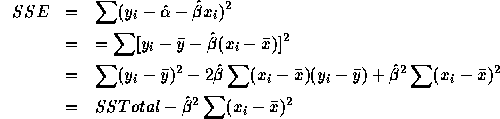Divide through by SSTotal and use the formula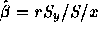where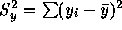, etc., to get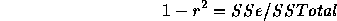Then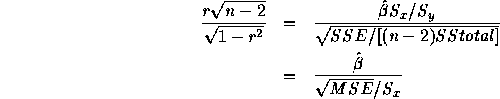which is the usual t-statistic. Note the use of the fact that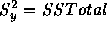.

Richard Lockhart
Fri Apr 3 22:09:46 PST 1998